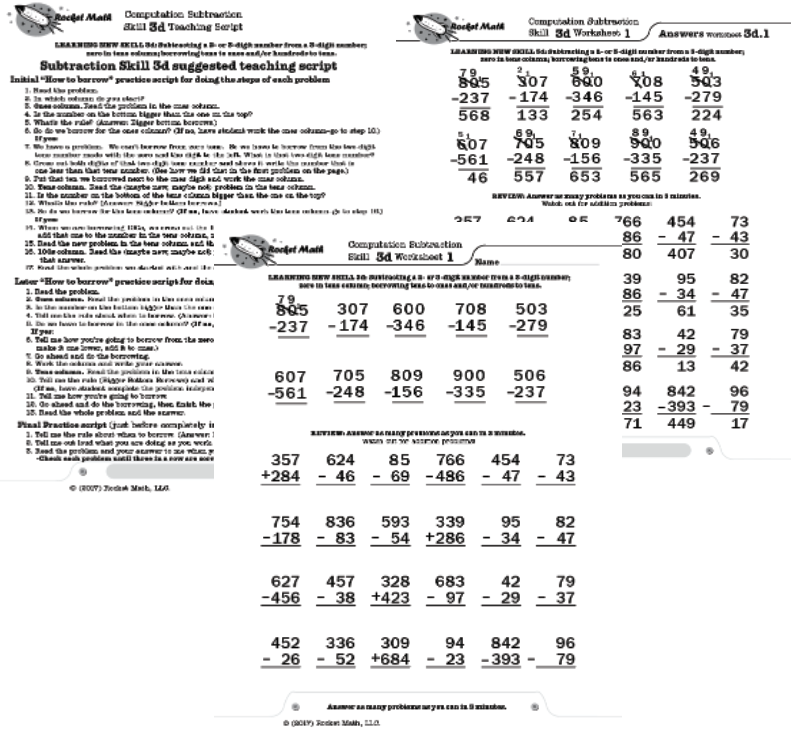## Teach how to do (compute) multi-step subtraction problems

After becoming fluent with subtraction facts, the best way for students to retain the knowledge of those facts is by doing subtraction computation.  If students have not been taught subtraction computation, Subtraction–Learning Computation breaks it down into 18 small, easy-to-learn steps that are numbered in a teaching sequence that leaves nothing to chance.

## Find out where to begin instruction

Even better the instructional materials include an assessment of all the skills in subtraction computation in order, so you can test the knowledge of the student(s) before beginning instruction to see where to start. You can use this assessment to find very specific “holes” in student skills and then have the exact problems and explanation to fill that hole.

## Skills taught in this Learning Track

Note that the number for each skill gives the grade level as well as indicating the teaching sequence.  Skill 3b is a 3rd grade skill and after skill 3g is learned the next in the sequence, skill 4a is best taught in fourth grade.  Minor changes have been made, but for the most part, the sequence of skills is drawn from M. Stein, D. Kinder, J. Silbert, and D. W. Carnine, (2006) Designing Effective Mathematics Instruction: A Direct Instruction Approach (4th Edition) Pearson Education: Columbus, OH.

(1b) Subtract from 2 digits; no renaming.

(2a) Subtract from 2digits; renaming required.

(2b) Subtract from 3 digits; borrow from 10s.

(3a) Subtract from 3 digits; borrow from 100s.

(3b) Subtract from 3 digits; borrow either place.

(3c) Subtract tens minus one facts.

(3d) Subtract from 3 digits; zero in 10s; borrow 10s or 100s.

(3e) Read and write thousands numbers, use commas.

(3f) Subtract from 4 digits; borrow from 1000s.

(3g) Subtract from 4 digits; borrow once or more.

(4a) Subtract from 4 digits; zero in 10s or 100s column

(4b) Subtract from 4 digits; zero in 10s column, 1 in 100s.

(4c) Subtract hundreds minus one facts.

(4d) Subtract from 4 digits; zero in 10s and 100s column.

(4e) Subtract 1, 2, or 3 digits from 1,000.

(4f) Subtract 5 and 6 digits with borrowing.

(5a) Subtract thousands minus one facts.

(5b) Subtract from a number with four zeroes.

## How to teach this Learning Track

For each skill there is a suggested Teaching Script giving the teacher/tutor/parent consistent (across all the skills we use the same explanation) language of instruction on how to do the skill.  My favorite part is the rule students are taught for when to borrow (often confusing for students): Bigger bottom borrows.  Simple, easy-to-remember and consistently correct.  The script helps walk the student through the computation process.  For the teacher, in addition to the script, there are answer keys for the five worksheets provided for each skill.

Each worksheet is composed of two parts.  The top has examples of the skill being learned that can be worked by following the script.  After working through those examples with the teacher the student is then asked to work some review problems of addition problems that are already known.  The student is asked to do as many as possible in 3 minutes—a kind of sprint.  If all is well the student should be able to do all the problems or nearly all of them, but finishing is not required.  Three minutes of review is sufficient for one day.

There are five worksheets for each skill.  Gradually as the student learns the skill the teacher/tutor/parent can provide progressively less help and the student should be able to do the problems without any guidance by the end of the five worksheets.  There are suggestions for how to give less help in the teaching scripts.